maths > construction-basics

Construction of Kite

what you'll learn...

overview

In this page, constructing kites is explained. It is outlined as follows.

•  Properties of kites is explained

•  The number of independent parameters in a kite is 1$1$

•  For a given parameter, construction of kites is approached as combination of triangles (sss, sas, asa, rhs, sal) and using the properties of kites.

Understanding Kite

A kite is a quadrilateral with two pair of equal and adjacent sides.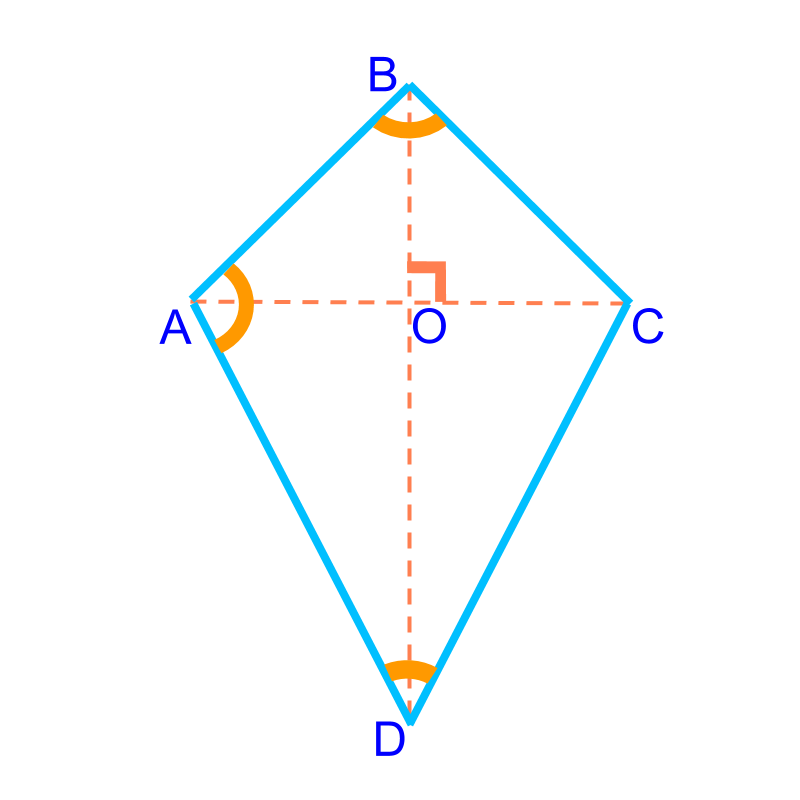A quadrilateral is defined by 5$5$ parameters. For a kite, the following properties provide additional dependency of parameters

•  two pair of equal sides

•  major diagonal perpendicularly bisects the minor diagonal. the diagonal that divides the kite into two congruent triangles is called major. The other diagonal is the minor diagonal.

•  major diagonal bisects the angles at the vertices

•  two equal opposite angles and two unequal opposite angles -- all sum up to 360${360}^{\circ}$

A kite is defined by 3$3$ parameters.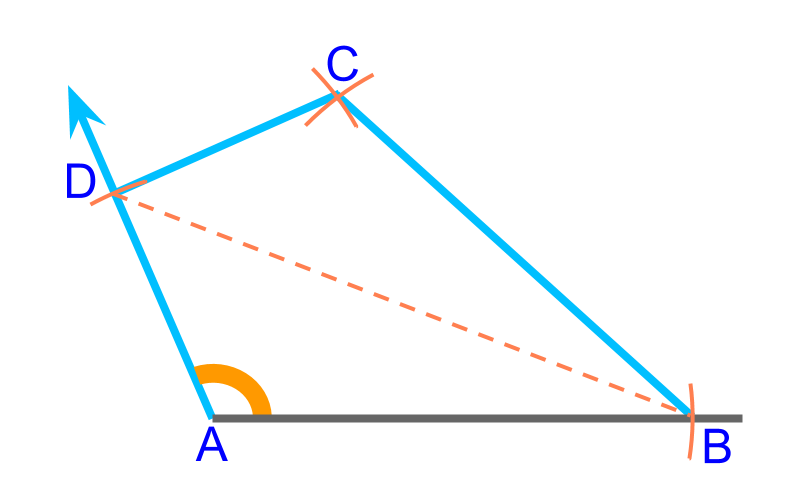To construct a kite, 2$2$ unequal sides (¯¯¯¯¯¯AB$\overline{A B}$, $\overline{B C}$) and the angle between them ($\angle B$) are given. This is illustrated in the figure. To construct, consider this as an SAS triangle in $B A D$ and SSS triangle $B C D$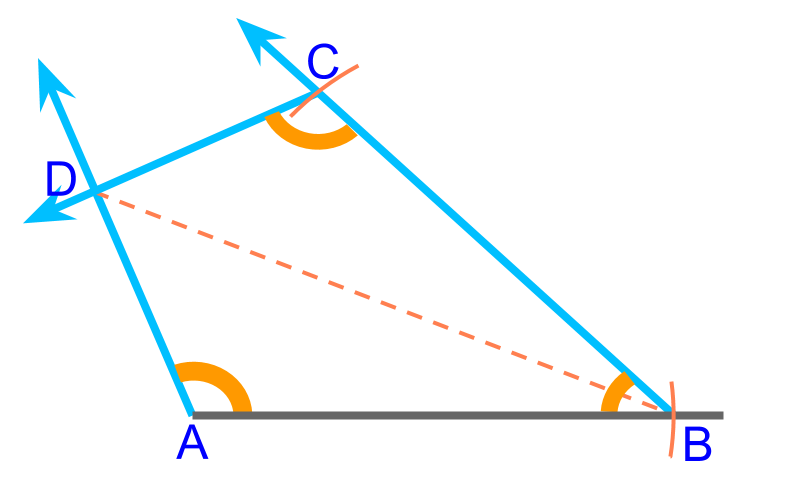To construct a kite, a side ($\overline{A B}$) and $2$ angles ($\angle A$,$\angle B$) are given. This is illustrated in the figure. To construct, consider as an SAS triangle in $A B C$ and ASA triangle $A C D$.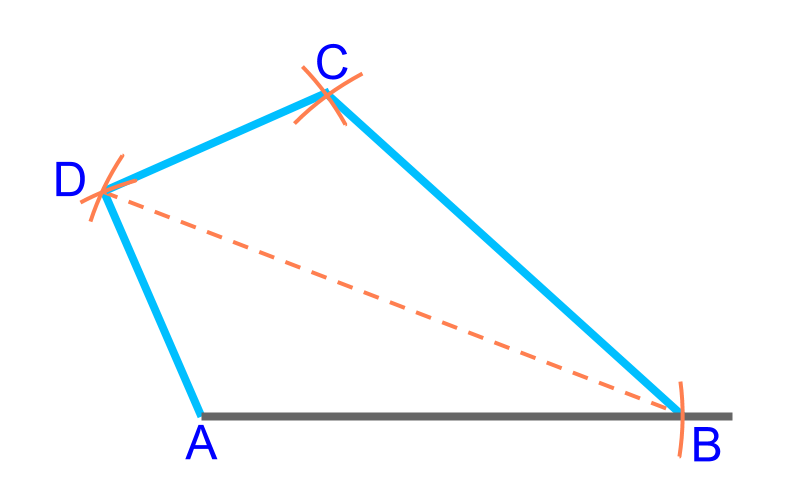To construct a kite, $2$ unequal sides ($\overline{A B}$, $\overline{B C}$) and the major diagonal ($\overline{B D}$) are given. This is illustrated in the figure. To construct, consider as two SSS triangles in $A B D$ and $B D C$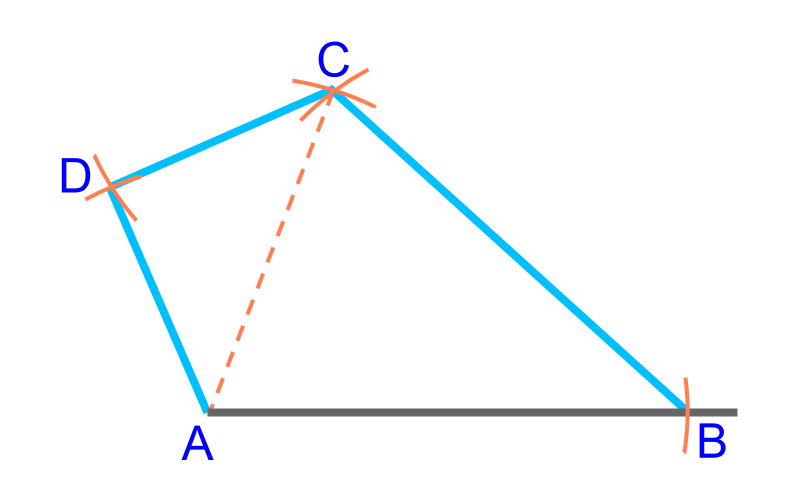To construct a kite, $2$ unequal sides ($\overline{A B}$, $\overline{B C}$) and the minor diagonal ($\overline{A C}$) are given. This is illustrated in the figure. To construct, consider as two isosceles SSS triangles in $A B C$ and $A C D$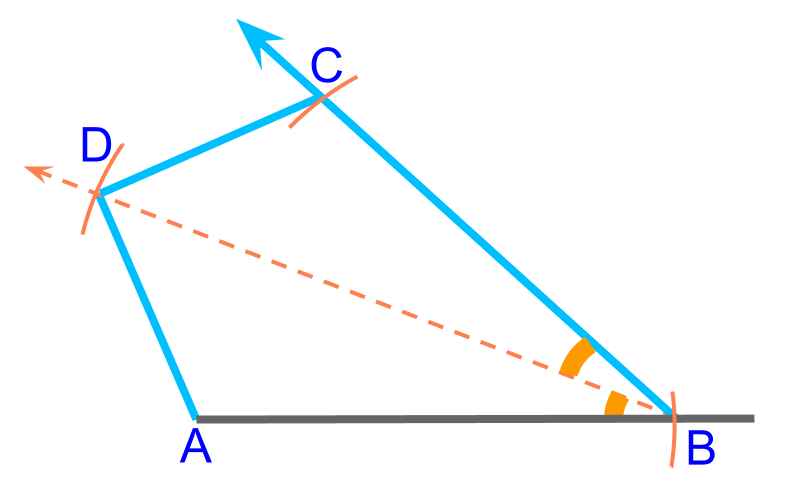To construct a kite, a side ($\overline{A B}$), the major diagonal ($\overline{B D}$), and angle ($\angle A B D$) between them are given. This is illustrated in the figure. To construct, consider this as two SAS triangles in $A B D$ and $D B C$

summaryConstruction of Kite :

Properties of Kite:

•  two pair of equal sides

•  major diagonal perpendicularly bisects the minor diagonal. The diagonal that divides the kite as two congruent triangles is the major diagonal.

•  major diagonal bisects the angles at the vertices

•  two equal opposite angles and two unequal opposite angles sum up to ${360}^{\circ}$

The formulations of questions

•  $2$ unequal sides and the angle between them

•  $1$ side and $2$ angles

•  $2$ unequal sides and the major diagonal

•  $2$ unequal sides and the minor diagonal

•  $1$ side, major diagonal and the angle between them

Outline